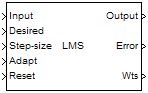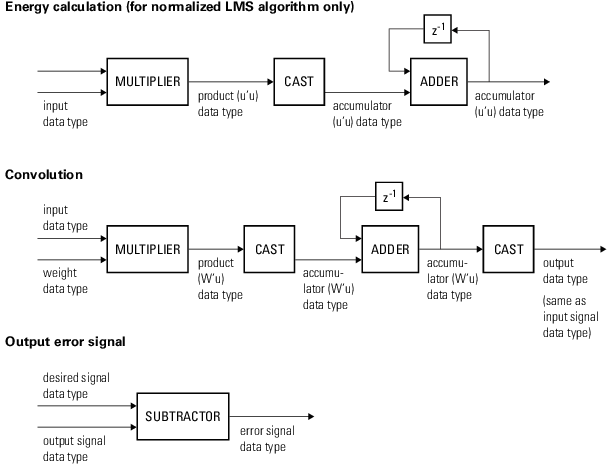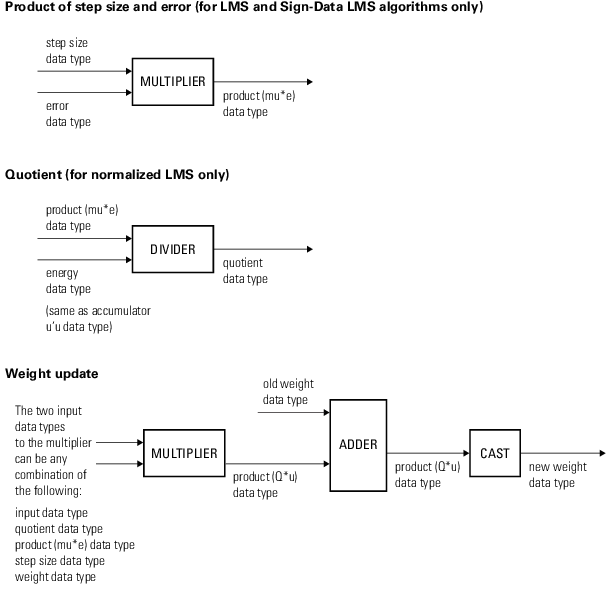Documentation

### This is machine translation

Mouseover text to see original. Click the button below to return to the English version of the page.

# LMS Filter

Compute output, error, and weights using LMS adaptive algorithm

## Library

`dspadpt3`## Description

The LMS Filter block can implement an adaptive FIR filter using five different algorithms. The block estimates the filter weights, or coefficients, needed to minimize the error, e(n), between the output signal y(n) and the desired signal, d(n). Connect the signal you want to filter to the Input port. The input signal can be a scalar or a column vector. Connect the desired signal to the Desired port. The desired signal must have the same data type, complexity, and dimensions as the input signal. The Output port outputs the filtered input signal, which is the estimate of the desired signal. The Error port outputs the result of subtracting the output signal from the desired signal.

When you select `LMS` for the Algorithm parameter, the block calculates the filter weights using the least mean-square (LMS) algorithm. This algorithm is defined by the following equations.

`$\begin{array}{c}y\left(n\right)={w}^{T}\left(n-1\right)u\left(n\right)\\ e\left(n\right)=d\left(n\right)-y\left(n\right)\\ w\left(n\right)=\alpha w\left(n-1\right)+f\left(u\left(n\right),e\left(n\right),\mu \right)\end{array}$`

The various LMS adaptive filter algorithms available in this block are defined as:

• LMS:

`$f\left(u\left(n\right),e\left(n\right),\mu \right)=\mu e\left(n\right){u}^{*}\left(n\right)$`

• Normalized LMS:

`$f\left(u\left(n\right),e\left(n\right),\mu \right)=\mu e\left(n\right)\frac{{u}^{\ast }\left(n\right)}{\epsilon +{u}^{H}\left(n\right)u\left(n\right)}$`

In Normalized LMS, to overcome potential numerical instability in the update of the weights, a small positive constant, ε, has been added in the denominator. For double-precision floating-point input, ε is 2.2204460492503131e-016. For single-precision floating-point input, ε is 1.192092896e-07. For fixed-point input, ε is 0.

• Sign-Error LMS:

`$f\left(u\left(n\right),e\left(n\right),\mu \right)=\mu \text{sign}\left(e\left(n\right)\right){u}^{*}\left(n\right)$`

• Sign-Data LMS:

`$f\left(u\left(n\right),e\left(n\right),\mu \right)=\mu e\left(n\right)\text{sign}\left(u\left(n\right)\right)$`

where u(n) is real.

• Sign-Sign LMS:

`$f\left(u\left(n\right),e\left(n\right),\mu \right)=\mu \text{sign}\left(e\left(n\right)\right)\text{sign}\left(u\left(n\right)\right)$`

where u(n) is real.

The variables are as follows:

VariableDescription

n

The current time index

u(n)

The vector of buffered input samples at step n

u*(n)

The complex conjugate of the vector of buffered input samples at step n

w(n)

The vector of filter weight estimates at step n

y(n)

The filtered output at step n

e(n)

The estimation error at step n

d(n)

The desired response at step n

µ

αThe leakage factor (0 < α ≤ 1)

ε

A constant that corrects any potential numerical instability that occurs during the update of weights.

Use the Filter length parameter to specify the length of the filter weights vector.

The Step size (mu) parameter corresponds to µ in the equations. For convergence of the normalized LMS equations, 0<µ<2. You can either specify a step size using the input port, Step-size, or by entering a value in the Block Parameters: LMS Filter dialog.

Enter the initial filter weights w(0) as a vector or a scalar in the Initial value of filter weights text box. When you enter a scalar, the block uses the scalar value to create a vector of filter weights. This vector has length equal to the filter length and all of its values are equal to the scalar value.

When you select the Adapt port check box, an Adapt port appears on the block. When the input to this port is greater than zero, the block continuously updates the filter weights. When the input to this port is less than or equal to zero, the filter weights remain at their current values.

When you want to reset the value of the filter weights to their initial values, use the Reset port parameter. The block resets the filter weights whenever a reset event is detected at the Reset port. The reset signal rate must be the same rate as the data signal input.

From the Reset port list, select `None` to disable the Reset port. To enable the Reset port, select one of the following from the Reset port list:

• `Rising edge` — Triggers a reset operation when the Reset input does one of the following:

• Rises from a negative value to a positive value or zero

• Rises from zero to a positive value, where the rise is not a continuation of a rise from a negative value to zero (see the following figure)• `Falling edge` — Triggers a reset operation when the Reset input does one of the following:

• Falls from a positive value to a negative value or zero

• Falls from zero to a negative value, where the fall is not a continuation of a fall from a positive value to zero (see the following figure)• `Either edge` — Triggers a reset operation when the Reset input is a `Rising edge` or `Falling edge` (as described above)

• `Non-zero sample` — Triggers a reset operation at each sample time that the Reset input is not zero

Select the Output filter weights check box to create a Wts port on the block. For each iteration, the block outputs the current updated filter weights from this port.

### Fixed-Point Data Types

The following diagrams show the data types used within the LMS Filter block for fixed-point signals; the table summarizes the definitions of variables used in the diagrams:

VariableDefinition

u

Input vector

W

Vector of filter weights

µ

Step size

e

Error

Q

Quotient, $Q=\frac{\mu \cdot e}{u\text{'}u}$

Product u'u

Product data type in Energy calculation diagram

Accumulator u'u

Accumulator data type in Energy calculation diagram

Product W'u

Product data type in Convolution diagram

Accumulator W'u

Accumulator data type in Convolution diagram

Product $\mu \cdot e$

Product data type in Product of step size and error diagram

Product $Q\cdot u$

Product and accumulator data type in Weight update diagram. 1

1The accumulator data type for this quantity is automatically set to be the same as the product data type. The minimum, maximum, and overflow information for this accumulator is logged as part of the product information. Autoscaling treats this product and accumulator as one data type.You can set the data type of the parameters, weights, products, quotient, and accumulators in the block mask. Fixed-point inputs, outputs, and mask parameters of this block must have the following characteristics:

• The input signal and the desired signal must have the same word length, but their fraction lengths can differ.

• The step size and leakage factor must have the same word length, but their fraction lengths can differ.

• The output signal and the error signal have the same word length and the same fraction length as the desired signal.

• The quotient and the product output of the u'u, W'u, $\mu \cdot e$, and $Q\cdot u$ operations must have the same word length, but their fraction lengths can differ.

• The accumulator data type of the u'u and W'u operations must have the same word length, but their fraction lengths can differ.

The output of the multiplier is in the product output data type if at least one of the inputs to the multiplier is real. If both of the inputs to the multiplier are complex, the result of the multiplication is in the accumulator data type. For details on the complex multiplication performed, see Multiplication Data Types.

## Dialog Box

### Main Tab

Algorithm

Choose the algorithm used to calculate the filter weights.

Filter length

Enter the length of the FIR filter weights vector.

Specify step size via

Select `Dialog` to enter a value for step size in the Block parameters: LMS Filter dialog. Select `Input port` to specify step size using the Step-size input port.

Step size (mu)

Enter the step size μ. Tunable (Simulink).

Leakage factor (0 to 1)

Enter the leakage factor, 0 < 1 – μα ≤ 1. Tunable (Simulink).

Initial value of filter weights

Specify the initial values of the FIR filter weights.

Select this check box to enable the Adapt input port.

Reset port

Select this check box to enable the Reset input port.

Output filter weights

Select this check box to export the filter weights from the Wts port.

### Data Types Tab

Rounding mode

Specify the rounding mode for fixed-point operations as one of the following:

• `Floor`

• `Ceiling`

• `Convergent`

• `Nearest`

• `Round`

• `Simplest`

• `Zero`

For more details, see rounding mode.

Saturate on integer overflow

When you select this parameter, the block saturates the result of its fixed-point operation. When you clear this parameter, the block wraps the result of its fixed-point operation. For details on `saturate` and `wrap`, see overflow mode for fixed-point operations.

Parameters

This parameter is visible if, for the Specify step size via parameter, you choose `Dialog`. Choose how you specify the word length and the fraction length of the leakage factor and step size:

• When you select ```Same word length as first input```, the word length of the leakage factor and step size match that of the first input to the block. In this mode, the fraction length of the leakage factor and step size is automatically set to the binary-point only scaling that provides you with the best precision possible given the value and word length of the coefficients.

• When you select ```Specify word length```, you can enter the word length of the leakage factor and step size, in bits. In this mode, the fraction length of the leakage factor and step size is automatically set to the binary-point only scaling that provides you with the best precision possible given the value and word length of the coefficients.

• When you select ```Binary point scaling```, you can enter the word length and the fraction length of the leakage factor and step size, in bits. The leakage factor and the step size must have the same word length, but the fraction lengths can differ.

If, for the Specify step size via parameter, you choose `Input port`, the word length of the leakage factor is the same as the word length of the step size input at the Step size port. The fraction length of the leakage factor is automatically set to the best precision possible based on the word length of the leakage factor.

Weights

Choose how you specify the word length and fraction length of the filter weights of the block:

• When you select ```Same as first input```, the word length and fraction length of the filter weights match those of the first input to the block.

• When you select ```Binary point scaling```, you can enter the word length and the fraction length of the filter weights, in bits.

Products & quotient

Choose how you specify the word length and fraction length of u'u, W'u, $\mu \cdot e$, $Q\cdot u$, and the quotient, Q. Here, u is the input vector, W is the vector of filter weights, μ is the step size, e is the error, and Q is the quotient, which is defined as $Q=\frac{\mu \cdot e}{u\text{'}u}$

• When you select ```Same as first input```, the word length and fraction length of these quantities match those of the first input to the block.

• When you select ```Binary point scaling```, you can enter the word length and the fraction length of these quantities, in bits. The word length of the quantities must be the same, but the fraction lengths can differ.

Accumulators

Use this parameter to specify how you would like to designate the word and fraction lengths of the accumulators for the u'u and W'u operations.

### Note

This parameter is not used to designate the word and fraction lengths of the accumulator for the $Q\cdot u$ operation. The accumulator data type for this quantity is automatically set to be the same as the product data type. The minimum, maximum, and overflow information for this accumulator is logged as part of the product information. Autoscaling treats this product and accumulator as one data type.

See Fixed-Point Data Types and Multiplication Data Types for illustrations depicting the use of the accumulator data type in this block:

• When you select ```Same as first input```, these characteristics match those of the input to the block.

• When you select ```Binary point scaling```, you can enter the word length and the fraction length of the accumulators, in bits. The word length of both the accumulators must be the same, but the fraction lengths can differ.

Lock data type settings against changes by the fixed-point tools

Select this parameter to prevent the fixed-point tools from overriding the data types you specify on the block mask.

## References

Hayes, M.H. Statistical Digital Signal Processing and Modeling. New York: John Wiley & Sons, 1996.

## Supported Data Types

PortSupported Data Types

Input

• Double-precision floating point

• Single-precision floating point

• Signed fixed point

Desired

• Must be the same as Input for floating-point signals

• Must be any signed fixed-point data type when Input is fixed point

Step-size

• Must be the same as Input for floating-point signals

• Must be any signed fixed-point data type when Input is fixed point

• Double-precision floating point

• Single-precision floating point

• Boolean

• 8-, 16-, and 32-bit signed integers

Reset

• Double-precision floating point

• Single-precision floating point

• Boolean

• 8-, 16-, and 32-bit signed integers

Output

• Must be the same as Input for floating-point signals

• Must be the same as Desired for fixed-point signals

Error

• Must be the same as Input for floating-point signals

• Must be the same as Desired for fixed-point signals

Wts

• Must be the same as Input for floating-point signals

• Obeys the Weights parameter for fixed-point signals

 LMS Update DSP System Toolbox RLS Filter DSP System Toolbox Block LMS Filter DSP System Toolbox Fast Block LMS Filter DSP System Toolbox